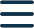# Public pension in Germany: Calculate your pension points

For each year that you work in Germany as an employee, you receive so-called pension points ("Rentenpunkte"), which are the basis for the amount of your public pension in retirement. To estimate how much your public pension is currently worth, you need to know the number of your accumulated points. Our pension point calculator estimates how many pension points you have collected so far.

## Let’s calculate whether you can save with a Pension Product

The current status of your pension points for your public pension in Germany can be estimated using the following key factors: Firstly, your monthly gross income
4.000 €
in the first year that you were working full-time in Germany, that is, in
2020 years
. And secondly your current monthly gross salary of
5.000 €
.

You have an estimated 4.03 of pension points, which at a current value of 36 € per point correspond to a total gross pension of 143 €.

### How is the public pension calculated in Germany?

The calculation of the public pension in Germany is based on the following formula:

Monthly pension = Pension points × Access factor × Current pension value × Pension type factor

This sounds very complicated, but it is actually quite simple:

#### Pension points ("Rentenpunkte" or "Entgeltpunkte")

If you work as an employee in Germany, you pay mandatory social contributions to the public pension insurance, among others. For every year that you pay into the pension fund, you receive pension points (“Rentenpunkte”). How many points you collect depends on the amount of your gross income. Exactly 1 pension point is given for the statistically calculated average income. In 2022, this will be 38,901 €. If you earn more or less, you also earn more or less points accordingly, i.e. 0,64 points for a gross annual income of 25.000 € and 1,8 points for 70.000 €. The maximum number of points you can collect annually is limited, in 2022 this limit is 2,17 points.

#### Access factor (“Zugangsfaktor”)

The access factor refers to the age at which you retire in relation to the standard retirement age. If you were born after 1966, the standard retirement age in Germany is 67. This means that starting your retirement at 67 leads to an access factor of 1. For each month you retire earlier, it decreases by 0,003 points. And for each month you retire later, there is an increase of 0,005 points. So if you work one year longer, your access factor is 1,06, if you are already retiring at 64 it is 0,892.

#### Pension value (“Rentenwert”)

The pension value is the value corresponding to one pension point. This value changes annually as it is adjusted to the overall economic situation. In 2022, it is 36,02 € for Western Germany and 35,52 € for Eastern Germany.

#### Pension type factor (“Rentenartfaktor”)

The pension type factor is the value that includes the specific form of the pension in the equation. For a regular old-age pension it is 1, for a partial or full disability, it is 0,5 or 1. Other possible pension types are the small and large widow(er)'s pension with factors of 0,25 and 0,5, respectively, and the half-orphan's and full orphan's pension with factors of 0,1 and 0,2.